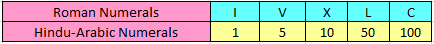# What are Roman Numerals | Rules and Examples | Maths Grade 4Rule 1 – Repetition of a Roman Numeral means addition.

• Only letters I, X, C, M can be repeated.
• No letter can be repeated more than 3 times.
• Letter V, L, D are never repeated.

Rule 2 – When a smaller numeral is placed on the left of the larger numeral, it means Subtraction.

• I can be subtracted from V and X only.
• X can be subtracted from L and C only.
• C can be subtracted from D and M only.

## Conversion of Hindu Arabic Numerals to Roman Numerals.

Question 1

Express 4 in Roman Numeral.

ExplanationStep I : Express the number in expanded form.
4 = 5 – 1

Step II : Convert Expanded form of number in Roman Numeral.
5 – 1 = (V) – (I) = IV

Hence, 4 would be represented as IV

Question 2

Express 12 in Roman Numeral.

ExplanationStep I : Express the number in Expanded form.
12 = 10 + 1 + 1

Step II : Convert Expanded form of number in Roman Numeral.
10 + 1 + 1 = (X) + (I) + (I)= XII

Hence, 12 would be represented as XII

Question 3

Express 48 in Roman Numeral.

ExplanationStep I : Express the number in Expanded form.
48   =      40    +    5      +   3
= (50 – 10) +   5      +   3

Step II : Convert Expanded form of number in Roman Numeral.
(50 – 10) + 5 + 3 = (L – X) + (V) + (III)= XLVIII

Hence, 48 would be represented as XLVIII

Question 4

Express 95 in Roman Numeral.

ExplanationStep I : Express the number in Expanded form.
95 =        90       +     5
=  (100 – 10)  +    5

Step II : Convert Expanded form of number in Roman Numeral.
(100 – 10) + 5 = (C – X) + V = XCV

Hence, 95 would be represented as XCV

## Conversion of Roman Numerals to Hindu Arabic Numerals.

Question 1

Express VI in Hindu Arabic Numeral.

ExplanationVI = V + I
= 5 + 1
= 6

Hence, VI can be represented as 6 in Hindu Arabic Numeral.

Question 2

Express XVII in Hindu Arabic Numeral.

ExplanationXVII = X + V + I + I
= 10 + 5 + 1 + 1
= 17

Hence, XVII can be represented as 17 in Hindu Arabic Numeral.

Question 3

Express XIV in Hindu Arabic Numeral.

ExplanationXIV = X + IV
= 10 + 4
= 14

Hence, Roman Numeral XIV can be represented as 14 in Hindu Arabic Numeral.

Question 4

Express XXVIII in Hindu Arabic Numeral.

ExplanationXXVIII = X + X + V + I + I + I
= 10 + 10 + 5 + 1 + 1 + 1
= 28

Hence, XXVIII can be represented as 28 in Hindu Arabic Numeral.